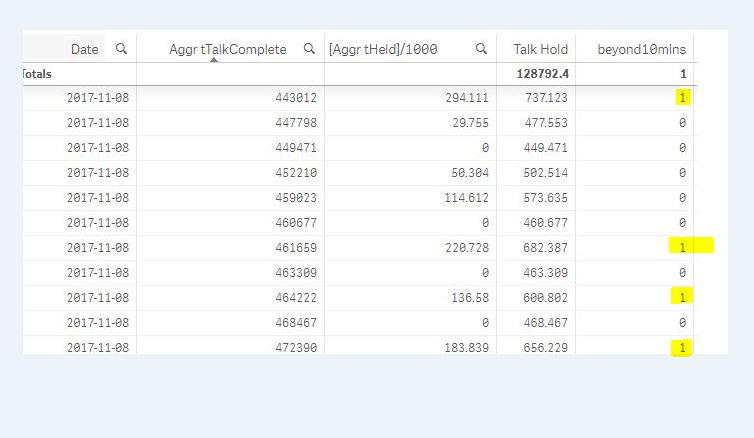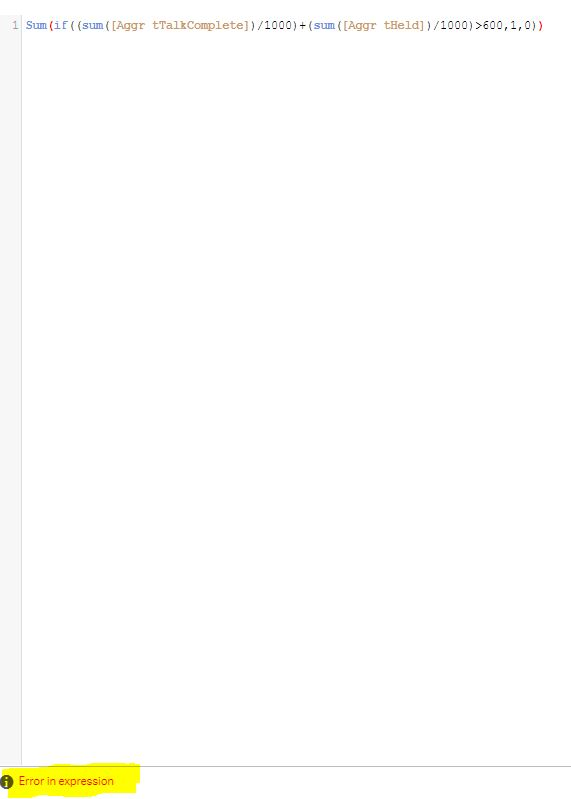# New to Qlik Sense

Discussion board where members can get started with Qlik Sense.

Announcements
Qlik® Product Spotlight: Discover what’s possible. Get more from our products.
See for yourself. Register today.
New Contributor III

## set analysis without variable panel

Hi Everyone,

I really need your expertise.

I'm thinking to create a variable for Talk + Hold.

vTalkHold = num(((sum([Aggr tTalkComplete]))/1000)+(((sum([Aggr tHeld]))/1000)),vFormatSeconds2)

then I will do the below expression in sheets. sum(if([vTalkHold]>600,1,0))

But I do not have a variable panel access so I have to directly have this measure in master Item.

1 Solution

Accepted Solutions
Honored Contributor III

## Re: set analysis without variable panel

Also you can use this

sum(aggr(if(((sum([Aggr tHeld])/1000)+(sum([Aggr tTalkComplete])/1000))>600,1,0),Date,[Aggr tHeld],[Aggr tTalkComplete]))

17 Replies
Honored Contributor III

## Re: set analysis without variable panel

Try,

sum(

if(

num(((sum([Aggr tTalkComplete]))/1000)+(((sum([Aggr tHeld]))/1000)),vFormatSeconds2) >600 ,1,0

)

)

New Contributor III

## Re: set analysis without variable panel

it says "error in expression"

New Contributor III

## Re: set analysis without variable panel

I have also tried this but still shows "error in expression"

sum(if(num(sum([Aggr tTalkComplete])/1000)+(sum([Aggr tHeld])/1000))>600,1,0))

Honored Contributor III

## Re: set analysis without variable panel

can you share sample app?

New Contributor III

## Re: set analysis without variable panel

Talk Hold = sum(([Aggr tTalkComplete])/1000)+(sum([Aggr tHeld])/1000)

beyond 10mins = if((sum([Aggr tTalkComplete])/1000)+(sum([Aggr tHeld])/1000)>600,1,0)so the problem is this.. the sumbeyond10mins should show 4Thank you Shraddha!Honored Contributor III

## Re: set analysis without variable panel

In 2nd Image showing Date & sumbeyond10mins what you have used?

Honored Contributor III

## Re: set analysis without variable panel

Sum(if((sum([Aggr tTalkComplete])/1000)+(sum([Aggr tHeld])/1000)>600,1,0))

Should work in 2nd image

New Contributor III

## Re: set analysis without variable panel

i just copied the expression of beyond10mins because i also tried this (exactly what you are suggesting) but says

error.Honored Contributor III

## Re: set analysis without variable panel

Try using Variables for

sum([Aggr tTalkComplete])/1000

and sum([Aggr tHeld])/1000

and use those variables here